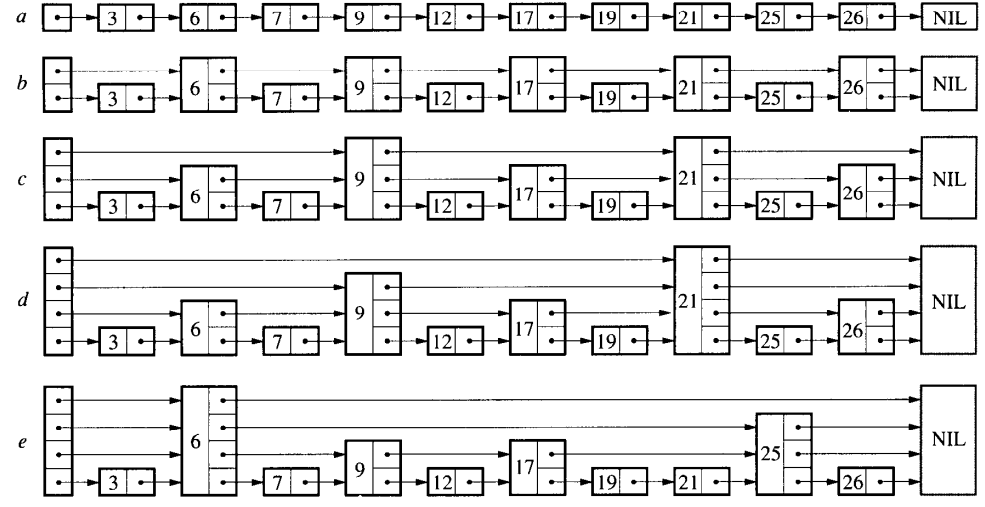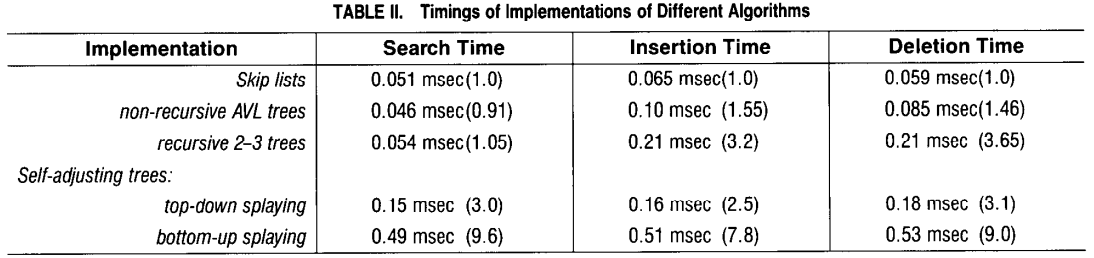# 凌云的博客

## skiplist 原理与实现

### skiplist 和 AVL

AVL 可用于表示如字典和有序列表等抽象数据类型。 当元素以随机顺序插入时，它们工作良好。 某些操作序列（例如按顺序插入元素）会产生执行效果非常差的效率。

skiplist 可能是替代 AVL 的一个不错的选择。 skiplist 通过随机数生成器来进行平衡。 尽管 skiplist 可能会出现糟糕的最坏性能，但没有任何输入序列能总是导致最坏的情况发生（非常类似于随机选择枢轴元素时的快速排序）。 skiplist 不太可能是显著不平衡的（例如，对于超过 250 个元素的字典，搜索将花费超过预期时间的三倍的机会小于百万分之一）。 skiplist 具有与通过随机插入构建的 AVL 类似的平衡属性，但不要求插入是随机的。

### skiplist### skiplist 算法

``````class Node(object):
def __init__(self, level, key, value):
self.forward = [None] * level
self.key = key
self.value = value
``````
• 其中 forward 是一个数组，包含了 n 个前向指针，数组的长度表示此节点的 level 值。
• key 用于对链表中的节点进行排序
• value 保存了节点的值，由于是 Python 实现的，value 可以保存任意类型的值

#### 初始化

``````class Skiplist(object):
def __init__(self, max_level = 32, p = 0.5):
self.max_level = max_level
self.current_level = 0
self.__probability = p
``````

#### 搜索

``````class Skiplist(object):
def search(self, key):
for level in range(self.current_level - 1, -1, -1):
while x.forward[level] is not None and x.forward[level].key < key:
x = x.forward[level]

x = x.forward
if x is not None and x.key == key:
return x.value
else:
return None
``````

#### 插入

``````class Skiplist(object):
def insert(self, key, value):
update = [None] * self.max_level
for level in range(self.current_level - 1, -1, -1):
while x.forward[level] is not None and x.forward[level].key < key:
x = x.forward[level]

update[level] = x

x = x.forward
if x is not None and x.key == key:
# found key, just update value
x.value = value
else:
new_level = self.__random_level()
if new_level > self.current_level:
for level in range(self.current_level, new_level):

self.current_level = new_level

x = Node(new_level, key, value)
for level in range(0, new_level):
x.forward[level] = update[level].forward[level]
update[level].forward[level] = x
``````

#### 删除

``````class Skiplist(object):
def delete(self, key):
update = [None] * self.max_level
for level in range(self.current_level - 1, -1, -1):
while x.forward[level] is not None and x.forward[level].key < key:
x = x.forward[level]

update[level] = x

x = x.forward
if x is not None and x.key == key:
for level in range(0, self.current_level):
if update[level].forward[level] != x:
break

update[level].forward[level] = x.forward[level]

del x
while self.current_level > 0 and\
``````

#### 随机选择节点的 level

``````class Skiplist(object):
def __random_level(self):
new_level = 1
while random.random() < self.__probability:
new_level += 1

return min(self.max_level, new_level)
``````

max_level 应该怎么选呢？这取决于我们选定的 p 值以及预期 skiplist 最多会容纳多少个节点，比如 p 为 1/2， skiplist 最多容纳 2^16 个节点，则可以将 max_level 设置为 16。

### 性能对比### 总结

• skiplist 和 AVL 有着类似的性能表现，但 skiplist 实现简单，逻辑直观
• 由于 skiplist 使用了随机算法来决定节点的 level，因此没有哪种插入顺序能总是导致 skiplist 出现最差性能
• skiplist 这是文中的 skiplist 的完整代码